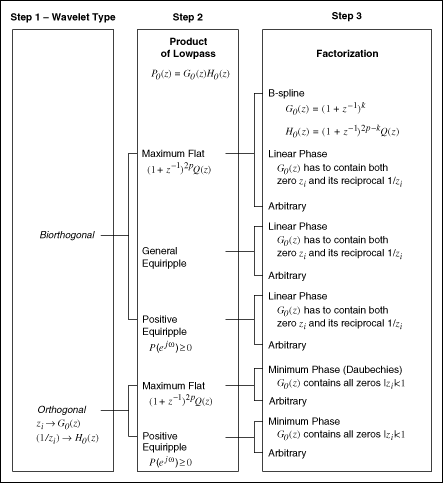# Interactively Designing Discrete Wavelets (Advanced Signal Processing Toolkit)

LabVIEW 2014 Advanced Signal Processing Toolkit Help

Edition Date: June 2014

Part Number: 372656C-01

»View Product InfoDownload Help (Windows Only)

Both the discrete wavelet transform and the inverse discrete wavelet transform are implemented using a set of cascaded two-channel perfect reconstruction (PR) filter banks.

The WA Wavelet Filter VI already contains a collection of predefined wavelets, including orthogonal wavelets (Haar, Daubechies, Coiflets, Symmlets) and biorthogonal wavelets (Biorthogonal, including FBI). You can apply the predefined wavelets directly to signal processing applications. If you cannot find a wavelet that best matches the signal, you can use the Wavelet Design Express VI to design a customized discrete wavelet.

The following figure shows the wavelet design process.Using the Wavelet Design Express VI, you need to complete the following steps to design wavelets:

1. Select the wavelet type.
2. Design the product of lowpass filters, P0(z), where the auxiliary function P0(z) is the product of G0(z) and H0(z).
3. Select the factorization type to factorize P0(z) into G0(z) and H0(z).

After you create an analysis lowpass filter G0(z) and a synthesis lowpass filter H0(z), the Wavelet Design Express VI automatically generates the corresponding analysis highpass filter G1(z) and synthesis highpass filter H1(z).

The design of discrete wavelets is essentially the design of two-channel PR filter banks. This book describes the steps that you can follow when using the Wavelet Design Express VI to design discrete wavelets and provides an example of designing the FBI wavelet.